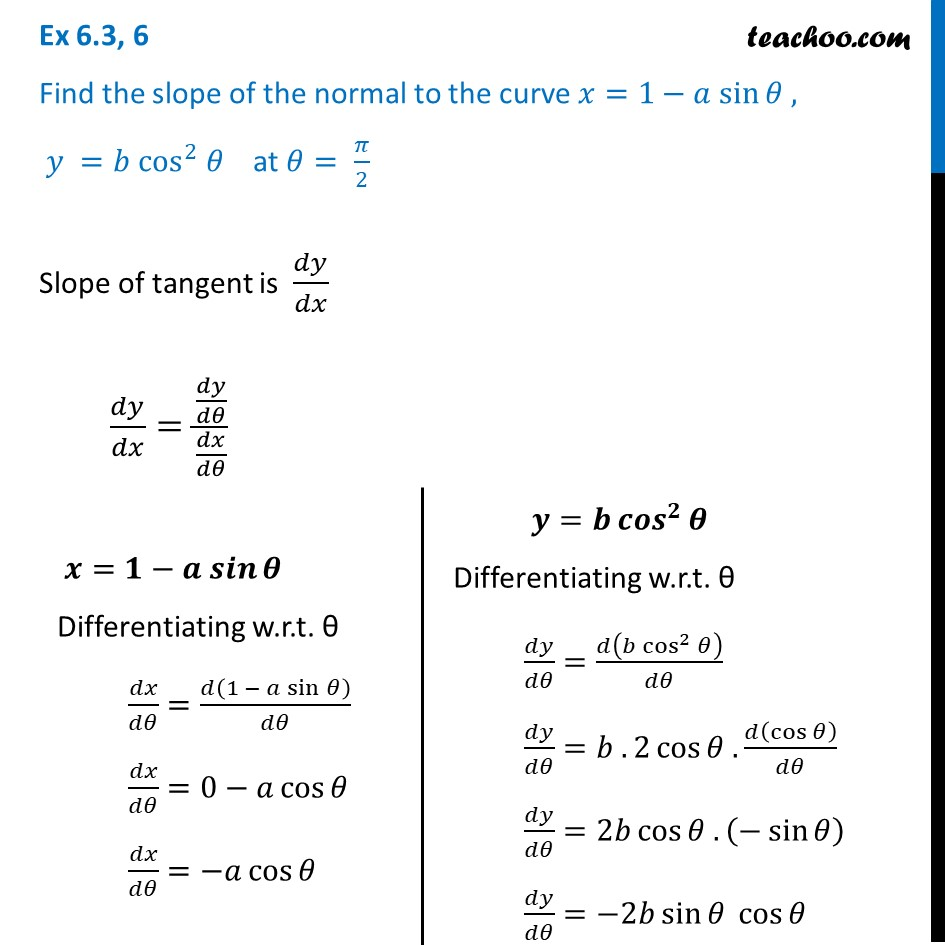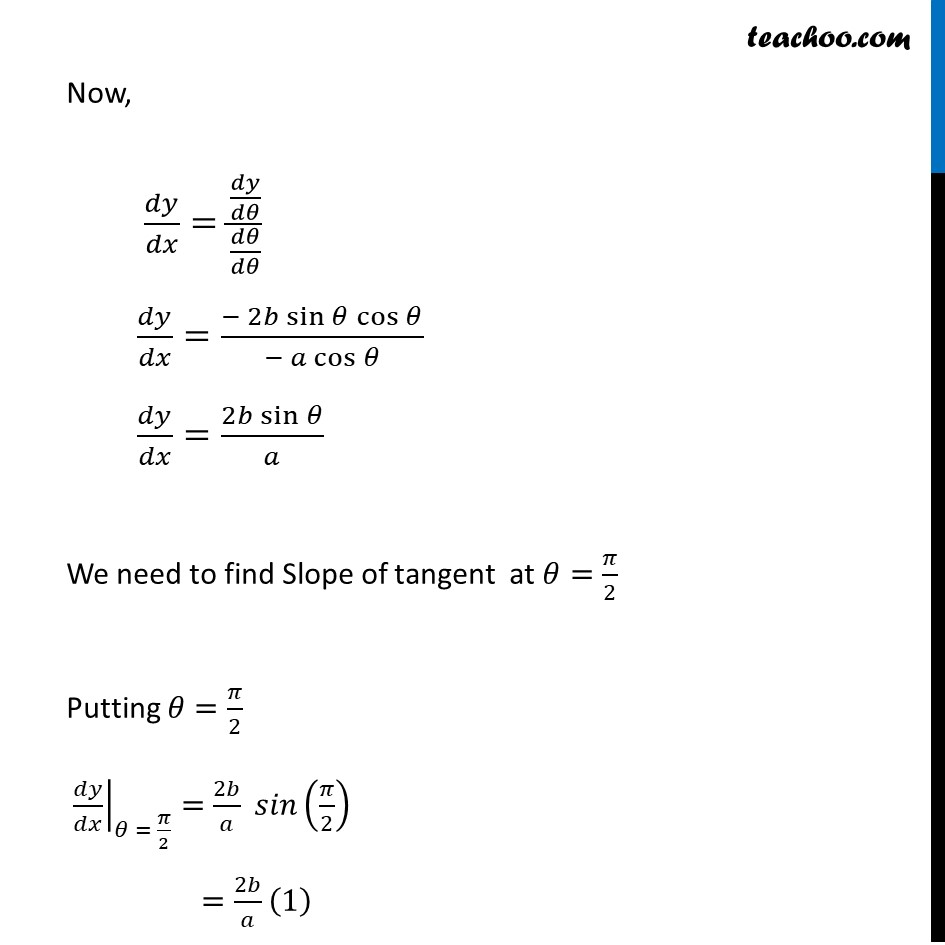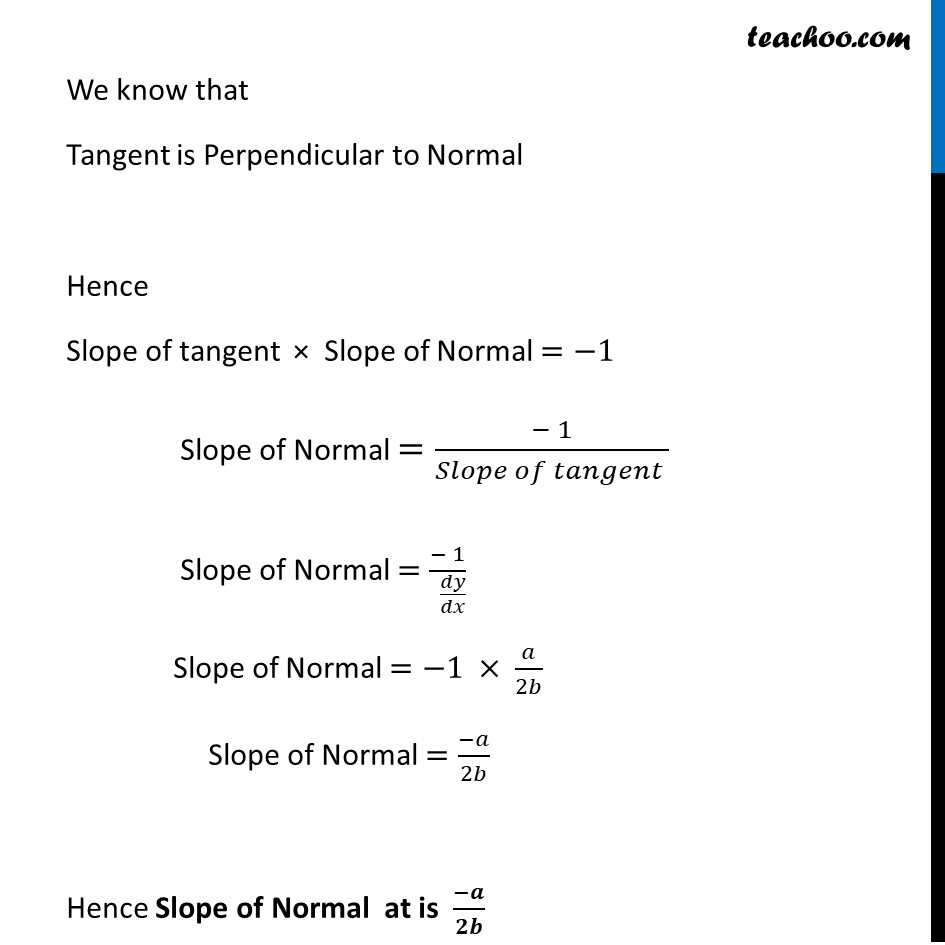Tangents and Normals (using Differentiation)

Chapter 6 Class 12 Application of Derivatives
Serial order wiseLearn in your speed, with individual attention - Teachoo Maths 1-on-1 Class

### Transcript

Question 6 Find the slope of the normal to the curve 𝑥=1−𝑎 sin⁡𝜃 , 𝑦 =𝑏 cos^2 𝜃 at 𝜃= 𝜋/2Slope of tangent is 𝑑𝑦/𝑑𝑥 𝑑𝑦/𝑑𝑥=(𝑑𝑦/𝑑𝜃)/(𝑑𝑥/𝑑𝜃) 𝒙=𝟏−𝒂 𝒔𝒊𝒏⁡𝜽 Differentiating w.r.t. θ 𝑑𝑥/𝑑𝜃=𝑑(1 − 𝑎 sin⁡𝜃 )/𝑑𝜃 𝑑𝑥/𝑑𝜃=0−𝑎 cos⁡𝜃 𝑑𝑥/𝑑𝜃=−𝑎 cos⁡𝜃 𝒚=𝒃 〖𝒄𝒐𝒔〗^𝟐⁡𝜽 Differentiating w.r.t. θ 𝑑𝑦/𝑑𝜃=𝑑(𝑏 cos^2⁡𝜃 )/𝑑𝜃 𝑑𝑦/𝑑𝜃=𝑏 . 2 cos⁡〖𝜃 . 𝑑(cos⁡𝜃 )/𝑑𝜃〗 𝑑𝑦/𝑑𝜃=2𝑏 cos⁡〖𝜃 . (−sin⁡𝜃 )〗 𝑑𝑦/𝑑𝜃=−2𝑏 sin⁡〖𝜃 cos⁡𝜃 〗 Now, 𝑑𝑦/𝑑𝑥=(𝑑𝑦/𝑑𝜃)/(𝑑𝜃/𝑑𝜃) 𝑑𝑦/𝑑𝑥=(− 2𝑏 sin⁡〖𝜃 cos⁡𝜃 〗)/(− 𝑎 cos⁡𝜃 ) 𝑑𝑦/𝑑𝑥=(2𝑏 sin⁡𝜃)/𝑎 We need to find Slope of tangent at 𝜃=𝜋/2 Putting 𝜃=𝜋/2 ├ 𝑑𝑦/𝑑𝑥┤|_(𝜃 = 𝜋/2)=2𝑏/𝑎 𝑠𝑖𝑛(𝜋/2) =2𝑏/𝑎 (1) We know that Tangent is Perpendicular to Normal Hence Slope of tangent × Slope of Normal =−1 Slope of Normal =(− 1)/(𝑆𝑙𝑜𝑝𝑒 𝑜𝑓 𝑡𝑎𝑛𝑔𝑒𝑛𝑡 ) Slope of Normal =(− 1)/( 𝑑𝑦/𝑑𝑥) Slope of Normal =−1 × 𝑎/2𝑏 Slope of Normal =(−𝑎)/2𝑏 Hence Slope of Normal at is (−𝒂)/𝟐𝒃